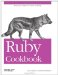# Adding Elements to a Hash

Problem

You have some items, loose or in some other data structure, which you want to put into an existing hash.

Solution

To add a single key-value pair, assign the value to the element lookup expression for the key: that is, call hash[key]=value. Assignment will override any previous value for that key.

```	h = {}
h["Greensleeves"] = "all my joy"
h # => {"Greensleeves"=>"all my joy"}
h["Greensleeves"] = "my delight"
h # => {"Greensleeves"=>"my delight"}
```

Discussion

When you use a string as a hash key, the string is transparently copied and the copy is frozen. This is to avoid confusion should you modify the string in place, then try to use its original form to do a hash lookup:

```	key = "Modify me if you can"
h = { key => 1 }
key.upcase! # => "MODIFY ME IF YOU CAN"
h[key] # => nil
h["Modify me if you can"] # => 1

h.keys # => ["Modify me if you can"]
h.keys.upcase!
# TypeError: can't modify frozen string
```

To add an array of key-value pairs to a hash, either iterate over the array with Array#each, or pass the hash into Array#inject. Using inject is slower but the code is more concise.

```	squares = [[1,1], [2,4], [3,9]]

results = {}
squares.each { |k,v| results[k] = v }
results # => {1=>1, 2=>4, 3=>9}

squares.inject({}) { |h, kv| h[kv] = kv; h }
# => {1=>1, 2=>4, 3=>9}
```

To turn a flat array into the key-value pairs of a hash, iterate over the array elements two at a time:

```	class Array
def into_hash(h)
unless size % 2 == 0
raise StandardError, "Expected array with even number of elements"
end
0.step(size-1, 2) { |x| h[self[x]] = self[x+1] }
h
end
end

squares = [1,1,2,3,4,9]
results = {}
squares.into_hash(results) # => {1=>1, 2=>3, 4=>9}

[1,1,2].into_hash(results)
# StandardError: Expected array with even number of elements
```

To insert into a hash every key-value from another hash, use Hash#merge!. If a key is present in both hashes when a.merge!(b) is called, the value in b takes precedence over the value in a.

```	squares = { 1 => 1, 2 => 4, 3 => 9}
cubes = { 3 => 27, 4 => 256, 5 => 3125}
squares.merge!(cubes)
squares # =>{5=>3125, 1=>1, 2=>4, 3=>27, 4=>256}
cubes # =>{5=>3125, 3=>27, 4=>256}
```

Hash#merge! also has a nondestructive version, Hash#merge, which creates a new Hash with elements from both parent hashes. Again, the hash passed in as an argument takes precedence.

To completely replace the entire contents of one hash with the contents of another, use Hash#replace.

```	squares = { 1 => 1, 2 => 4, 3 => 9}
cubes = { 1 => 1, 2 => 8, 3 => 27}
squares.replace(cubes)
squares # => {1=>1, 2=>8, 3=>27}
```

This is different from simply assigning the cubes hash to the squares variable name, because cubes and squares are still separate hashes: they just happen to contain the same elements right now. Changing cubes won't affect squares:

```	cubes = 64
squares # => {1=>1, 2=>8, 3=>27}
```

Hash#replace is useful for reverting a Hash to known default values.

```	defaults = {:verbose => true, :help_level => :beginner }
args = {}
requests.each do |request|
args.replace(defaults)
request.process(args) #The process method might modify the args Hash.
end
```

• Recipe 4.12, "Building Up a Hash Using Injection," has more about the inject method
• Recipe 5.1, "Using Symbols as Hash Keys," for a way to save memory when constructing certain types of hashes
• Recipe 5.5, "Using an Array or Other Modifiable Object as a Hash Key," talks about how to avoid another common case of confusion when a hash key is modifiedRuby Cookbook (Cookbooks (OReilly))
ISBN: 0596523696
EAN: 2147483647
Year: N/A
Pages: 399